### Home > CAAC > Chapter 13 > Lesson 13.OF1-S > Problem7-136

7-136.
1. If f(x) = x4 and g(x) = 3(x + 2), find the value of each expression below. Homework Help ✎

1. f(2)

2. g(2)

3. f(g(2))

4. g(f(2))

5. Are f(x) and g(x) inverses of each other? Justify your answer.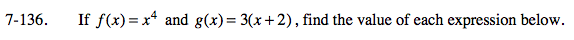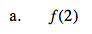16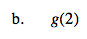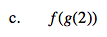Substitute the output for g(2) into f(x).

20,736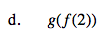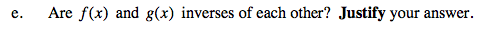What happens to the original input when the two functions are inverses?